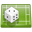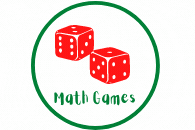# Math Games Fun Exercises for Seventh 7th GradeMoney and Price List – Basketball GamePatterns – Arithmetic, Geometric Progressions, Multiple Choice QuizSpelling Numbers – memory GameTime Units – memory GameRoman Numerals Game – Football GameMultiplication memory gameMultiplication Game- walk the plank gameMultiplication Sentences – Basketball GameOrdinal numbers memory game 1ordinal numbers memory game 2place value memory gamePlace value – walk the plank gameSpelling numbers above a thousand – memory gameSubtraction memory gameComparisons Game- En Garde Duel GameGeometry Game- Fing the teacher gameMissing Number Game -Penalty shoot gameSubtraction Game 1 -10- En gard duel gameSubtraction Game 1 – 10 – Fling the teacher gameSubtraction Game – Basketball gameSubtraction Game – Fling the teacherTelling the time game – memory gameNumber Spelling Game – Memory GameNumber Spelling Game – Walk the plank game

Fun Games for Math PracticeThis exercise will help kids practice Math in a fun way. Kids related to games very well. From preschool / kindergarten, first grade to sixth graders levels of math games. There are games for the following topics:

• Games to practice Addition, subtraction, Geometry, Comparison, Algebra, Shapes, Time, Fractions, Decimals, Sequence, Division, Metric system, Logarithms, ratios, probability, multiplication and more>>

The games include among other : memory games, Walk the plank, Fling the Teacher, En Garde Duel, Basketball Game, Penalty Shoot and more.

Website General Content:

This site is all about the following: math, maths, for kids, children, math activities for children,math for kids,kindergarten math,elementary math, first grade math, math games, worksheets, printables, exercises, preschool children, free online exercises, free, downloads, printable PDF, addition, subtraction, geometry, shapes, position, fractions, multiplication, division, arithmetic, algebra etc.

math activities for children, maths for kids, math games and exercises, math worksheets, printables, online, interactive, quizzes, for kindergarten, preschool, first grade, math practice, for teachers and parents, teach your kids math, help kids learn maths

How to get better at math,
How to get good at math,
why is math so hard,8th grade math tutoring,kids math tutor,math tutor for kids,7th grade math tutoring,math tutoring for kids,
math apps for kids,
free math websites, how to get better at math fast,how to become good at math overnight,
math made easy,how to understand maths easily,
how to get better at math for adults,
how to become good at math overnight,
how to be good at math,
how to get good at math again,
how to get good at math reddit,
how to understand maths easily,
math games,